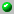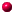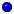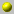the following papers are available on our server= dvi format= ps format= tex format= pdf formatdifference_elliptic_operators_and_root_systemsdifference_macdonald_mehta_conjectureelliptic_quantum_many_body_problem_and_double_affine_knizhnik_zamolodchikov_equation
induced_representations_of_double_affine_hecke_algebras_and_applicationsintertwining_operators_of_double_affine_hecke_algebraskadells_two_conjectures_for_macdonald_polynomialsmacdonalds_evaluation_conjectures_and_difference_fourier_transformmacdonalds_evaluation_conjectures_and_difference_fourier_transformmacdonalds_evaluation_conjectures_difference_fourier_transform_and_applicationsnon_symmetric_macdonalds_polynomials
non_symmetric_macdonalds_polynomials

Go back to the directory of pre and reprints.
Or search the database by keywords
Or look for the newest entires in the database